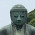## 2011/04/29

### Easy way to get yield curve : what you need is only "FRBData" package !

I made FRBData package and registerd it on CRAN.
This package allow you to download financial data from FRB's website.
This website provide many economical data such as consumer credit, money stock.

This article show you how to use this package.
(But, it has only a function about interest rate now.　I will create other functions to download other macro-economical data in next version.)

First, because some mirror-site don't update this package at this time, you should install it via CRAN.
(you may need to install the R which has version more than 2.12.)

`install.packages("FRBData", repos = "http://cran.r-project.org")`

Created by Pretty R at inside-R.org

Load library.
`library("FRBData")`

Created by Pretty R at inside-R.org

As an example, we download treasury and swap curve from 2005 to 2011.
```start <- as.Date("2005-12-31")
end   <- as.Date("2011-03-31")```
```curve.treasury <- GetInterestRates("TCMNOM", from = start, to = end)
curve.swap     <- GetInterestRates("SWAPS",  from = start, to = end)```

Created by Pretty R at inside-R.org

these data are an xts object.
```> class(curve.swap)
 "xts" "zoo"
> head(curve.swap)
1Y   2Y   3Y   4Y   5Y   7Y  10Y  30Y
2006-01-03 4.83 4.81 4.80 4.82 4.83 4.86 4.90 5.05
2006-01-04 4.79 4.76 4.76 4.78 4.80 4.84 4.90 5.08
2006-01-05 4.79 4.76 4.76 4.77 4.79 4.83 4.89 5.06
2006-01-06 4.81 4.78 4.78 4.80 4.81 4.85 4.90 5.08
2006-01-09 4.81 4.77 4.77 4.78 4.80 4.83 4.88 5.05
2006-01-10 4.83 4.81 4.80 4.82 4.83 4.86 4.92 5.08```
```
```
Created by Pretty R at inside-R.org

plot "10Y swap spread"
```#plot 10Y swap spread
plot(curve.swap[, "10Y"] - curve.treasury[, "10Y"], main = "swap spread")```
```
```
Created by Pretty R at inside-R.org

plot time-varying treasury term structure
```#plot yield curve by each year
curve.treasury.each.year <- t(curve.treasury[endpoints(curve.swap, on = "years")])
par(xaxt="n")
matplot(curve.treasury.each.year, main = "time-varying treasury yield curve",
type = "l", lwd = 2,lty = 1, xlab = "Term", ylab = "Yield")
legend(8.5, 2.0, legend = substr(colnames(curve.treasury.each.year), 1, 4),
col=1:ncol(curve.treasury.each.year), lty = 1, lwd = 2)
par(xaxt = "s")
axis(1,1:length(rownames(curve.treasury.each.year)),rownames(curve.treasury.each.year))```

Created by Pretty R at inside-R.org

If you would like to get other interest rate data, please check Reference manual.

I think that this package will provide you good chance of analyzing yield curve such as yield curve e arbitrage.
Enjoy !
(...and tell me interesting quant job in secret :) )

#### 2 件のコメント:

1.Seems this is gone now?

2.Thank you for your comment.

I decided to quit maintaining this package because there is better alternatives packages
- quantmod
- quandl